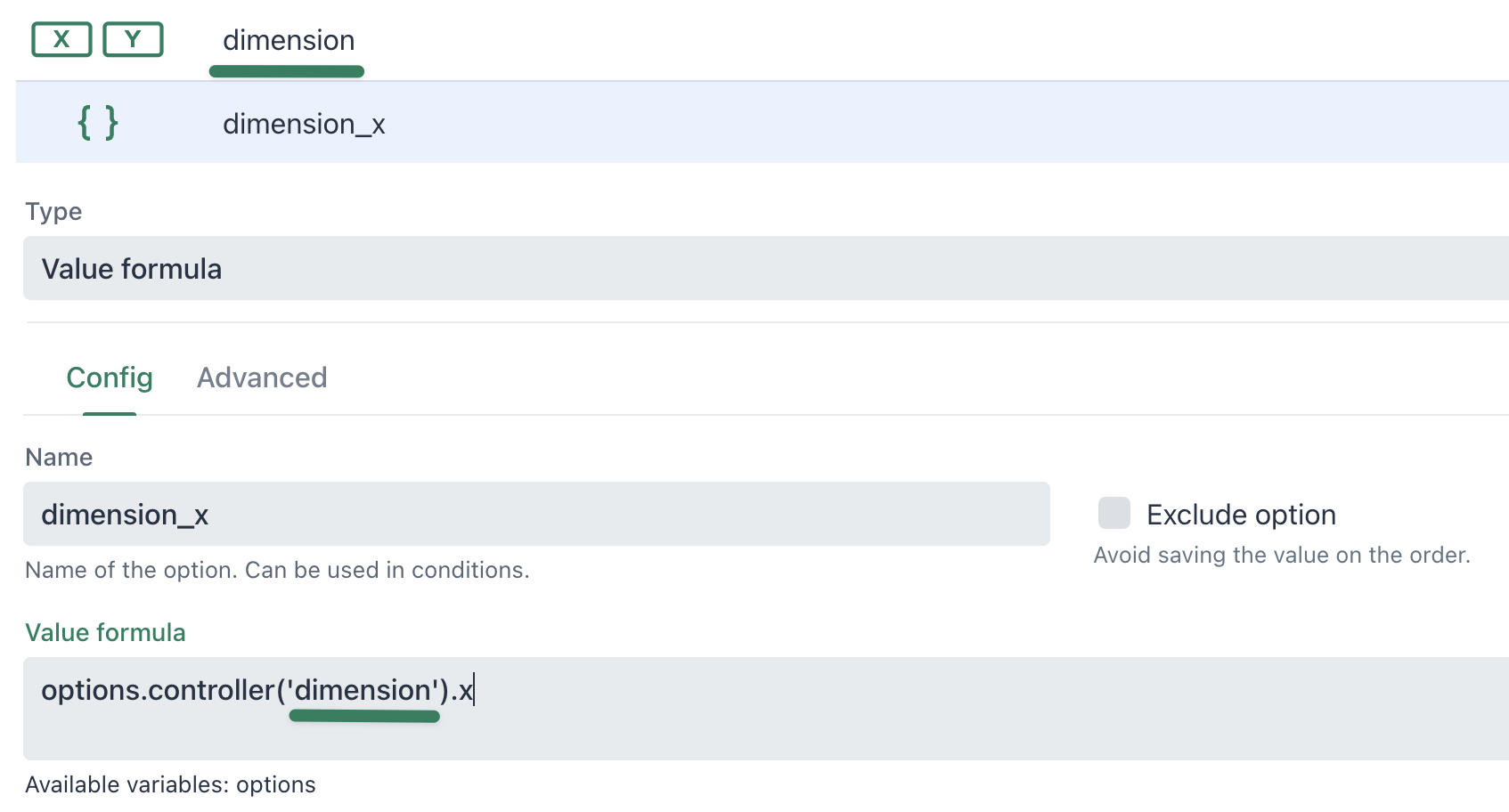# Price formula

## Enable extension

To enable the  Formula extension please visit: How can I add an Extension?

## How does the extension work?

The extension adds the ability to assign a javascript based price formula behind every option value.  When an option is active the app executes the javascript code to evaluate it's current price.

The price formula can access other option values and any global javascript values to calculate a custom price.

### Setup

Every option that can have a price, can also use a custom price formula to calculate its final price.

To add a price formula, click on the curly brackets icon in the price field: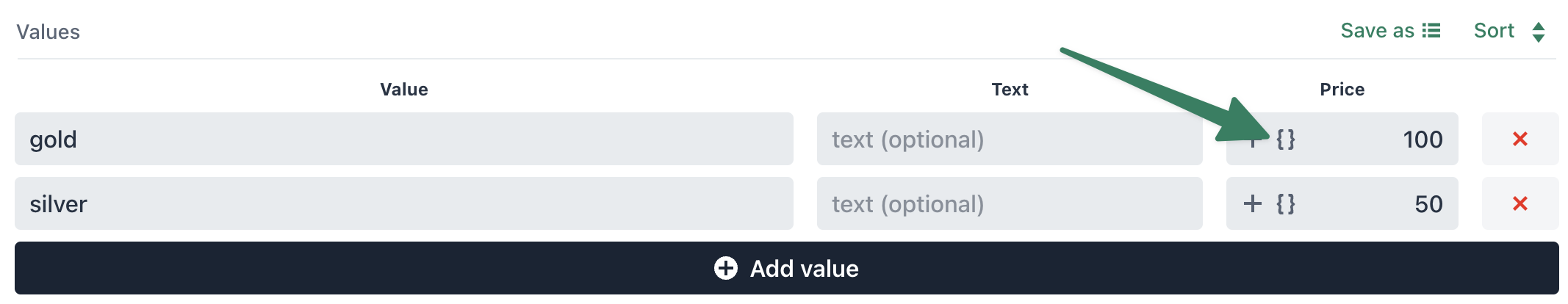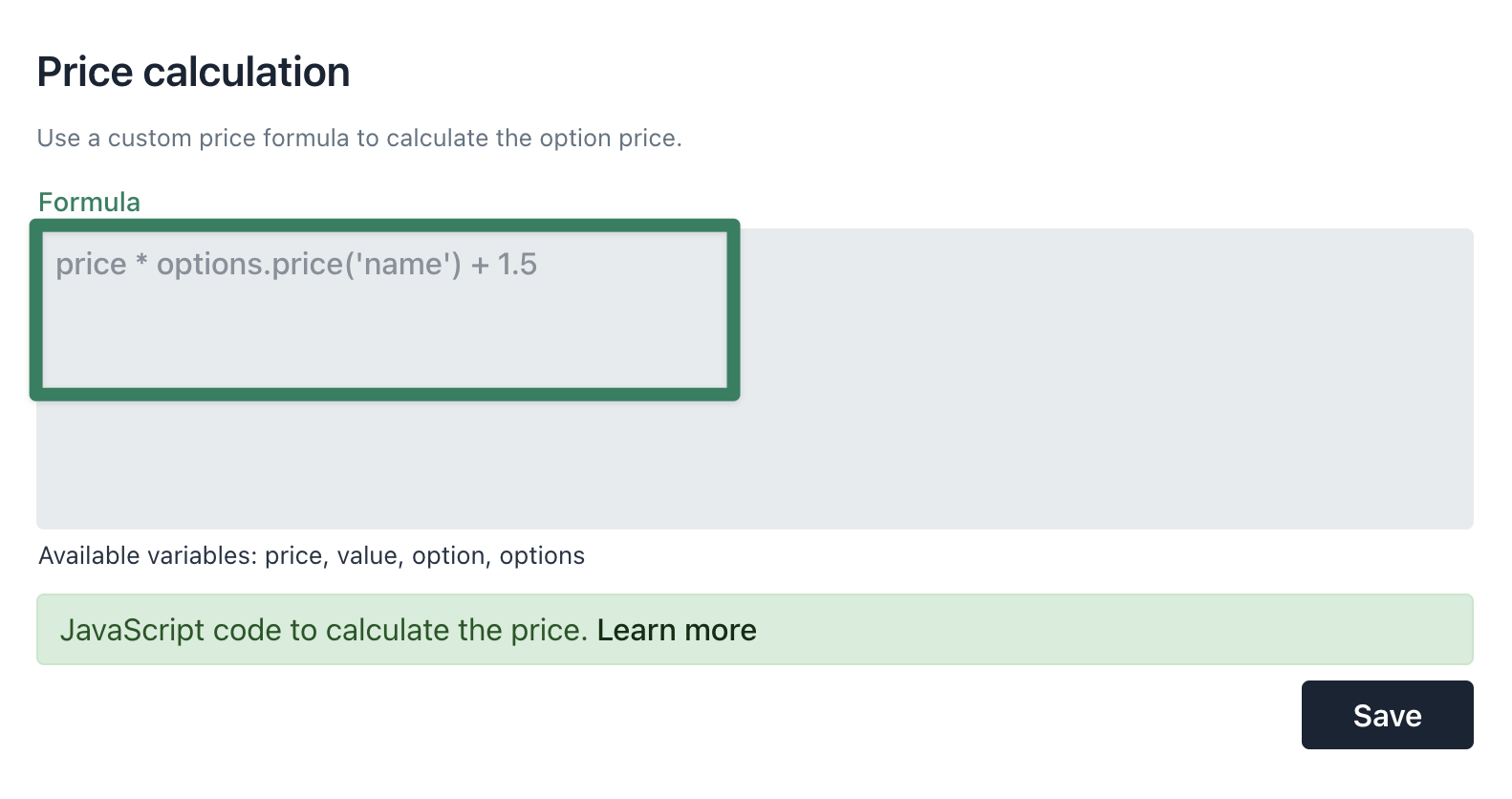When a price formula is used, the curly brackets icon will be highlighted in green: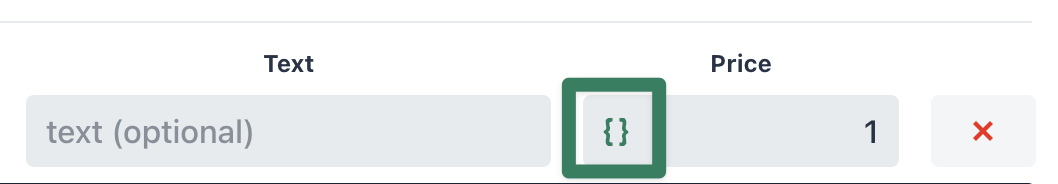### Available variables

The following variables are available in the price formula context:

#### price

The price variable contains the configured price of each option value.  The price can be used as a base for the final price calculation.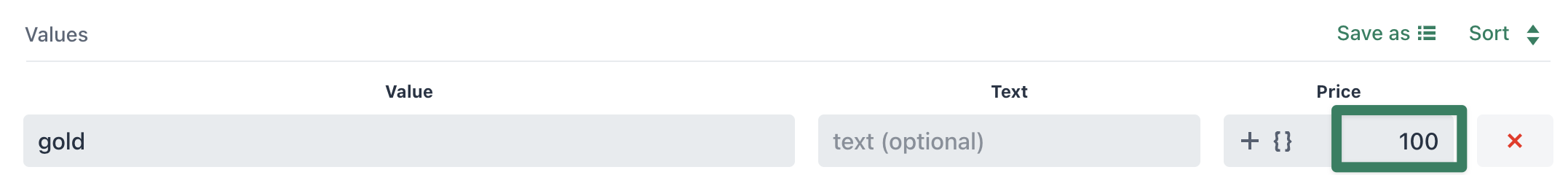#### value

The value variable contains the configured option value. The value can also be a number, this number could then also be used in price calculations.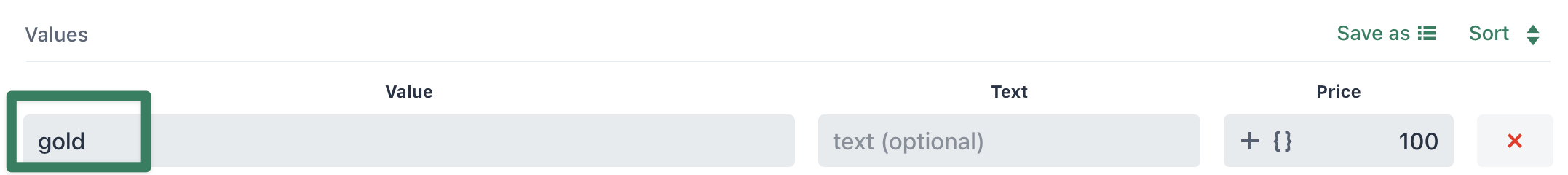#### option

The option variable is a reference to the full option object.  option.value contains the current selected option value.

#### options

The options variable is a helper object to access other options and their values/prices.

Every method on the object expects the option name as parameter: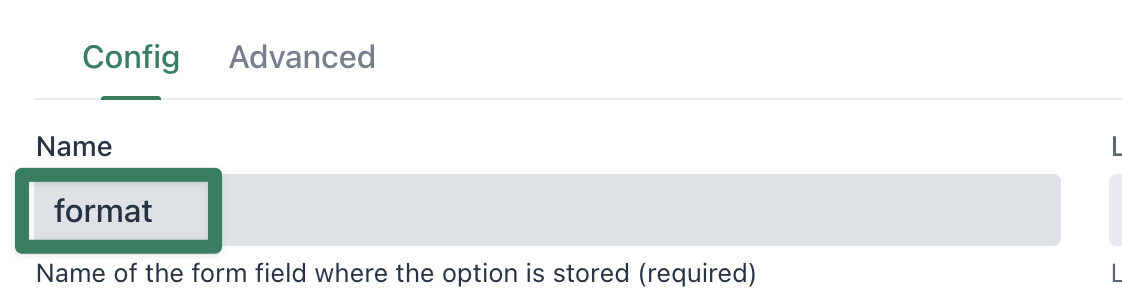options.value('format') Get the selected value of the option options.price('format') Get the current price of the option options.option('format') Get the full option reference options.controller('format') Get the option controller reference (only for advanced options like upload/dimension) options.variant() Get the current select variant object (useful to access the price or the variant title). options.weight('format', weight) Update the weight of the option (requires weight calculation to be enabled)

#### Price formula types

Price formulas can be one of the following types.

#### Expression

A price formula expression consists of a single line of Javascript containing a calculation:

`price * options.value('number')`

Here the price is calculated in combination with the selected value of the "number" option.

#### Function

A price formula function can consist of multiple lines of Javascript which supports declaring variables and using control flow (if/else/..) statements:

`// read the selected materialvar material = options.value('material');// calc price based on selectionif(material == 'gold'){ return price + 100;} else if(material == 'silver'){ return price + 50;} else { return price;}`

Price functions are useful for more complex calculations with multiple inputs and conditions on other options.

Important:  functions always require a return instruction.

### Examples

The following are some examples of possible uses of price formulas.

#### Price number calculation

For a dynamic price based on an entered number in a number option.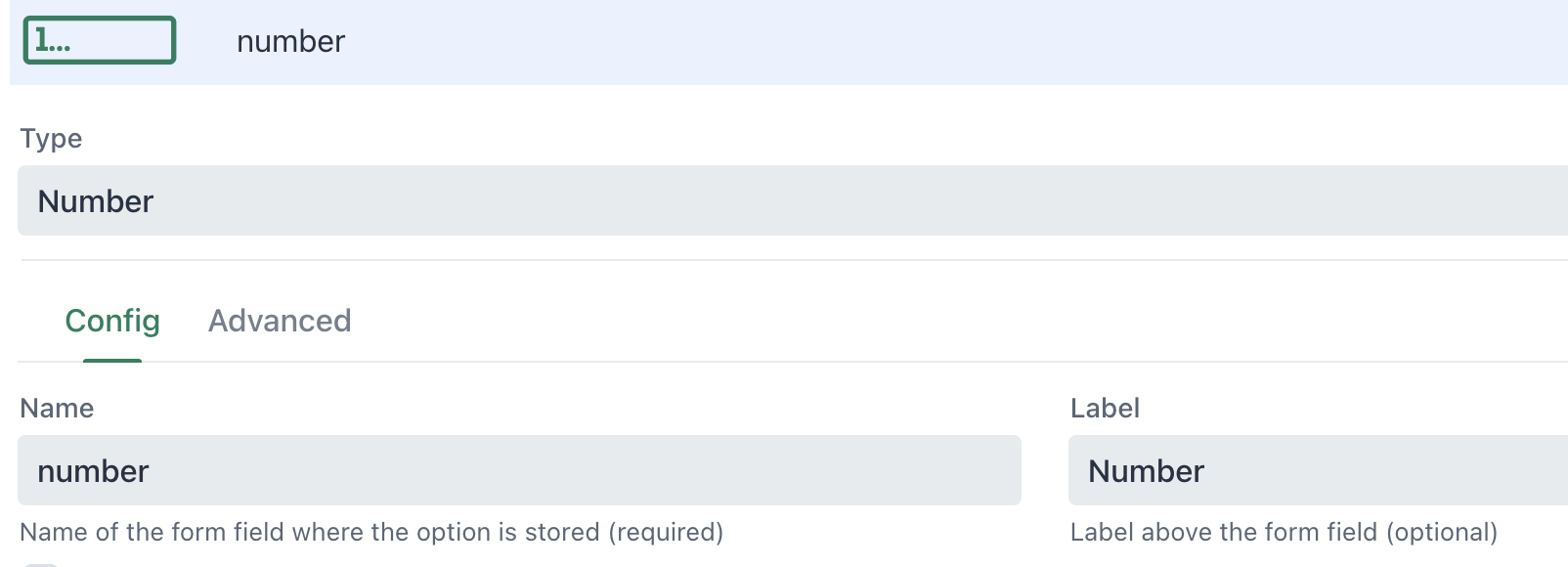Checkbox option with price formula: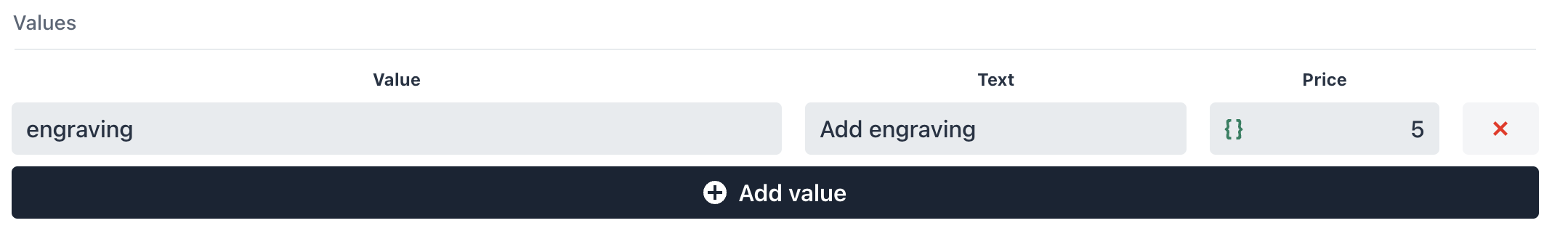`price * options.value('number')`

The engraving price automatically updates based on the entered number: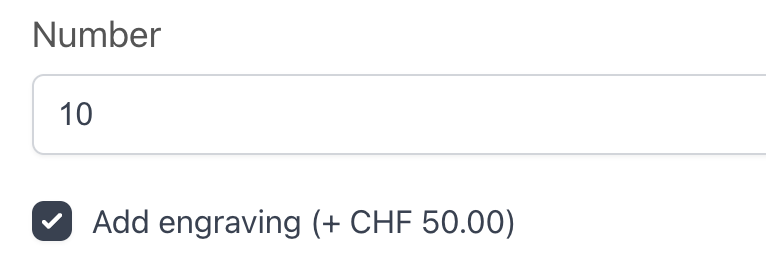#### Price based on different option selection

Changing the price of an option based on the selection of another option can simplify the option pricing setup for more complex requirements.

In this example, the size option contains three different sizes for a canvas image: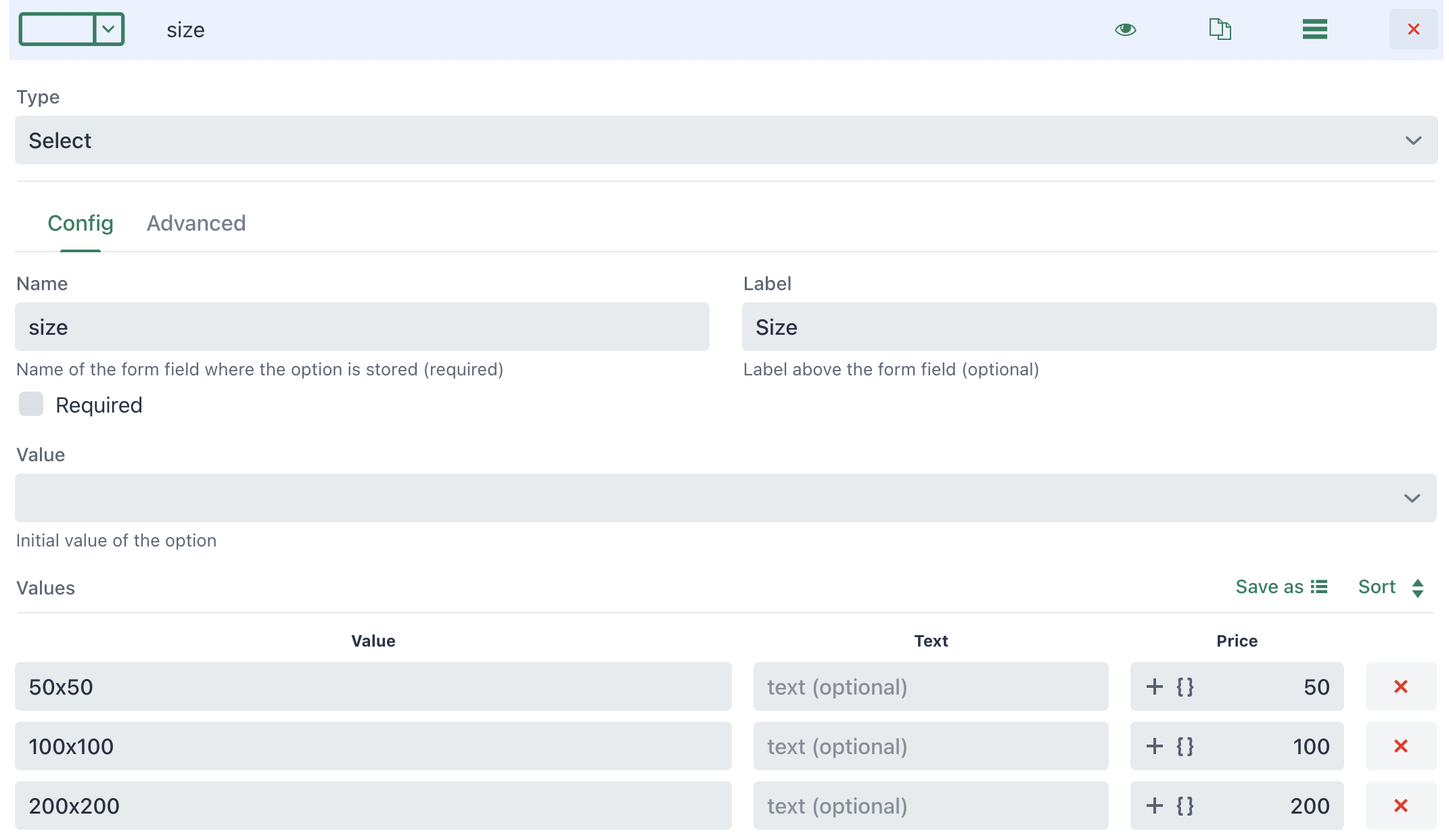The frame option lets the customer select if a framing should be added: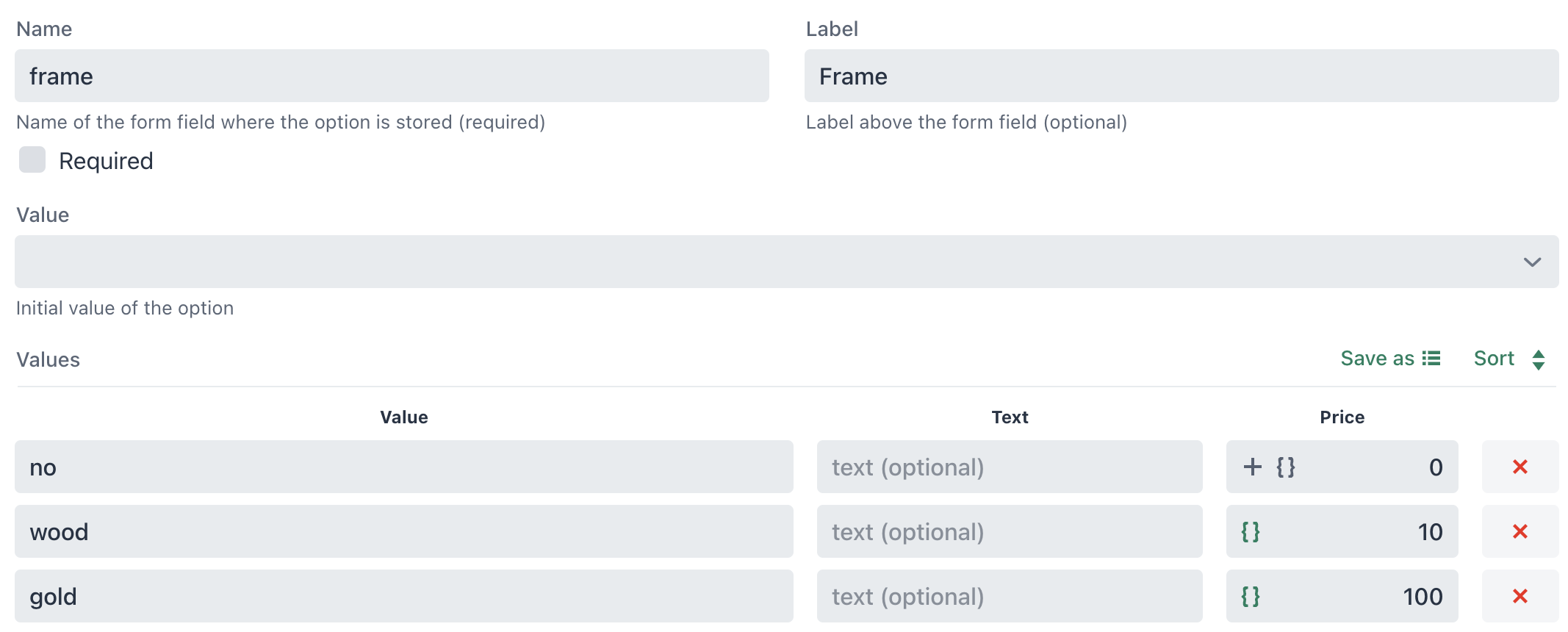`// read the selected sizevar size = options.value('size');// calc price based on sizeif(size == '100x100'){ return price * 2;} else if(size == '200x200'){  return price * 4;} else {  // default selection  return price; }`

Note: the price formula is identical, as the price variable is used to calculate the right price increase.

Now, depending on the selected size, the frame option price will be different: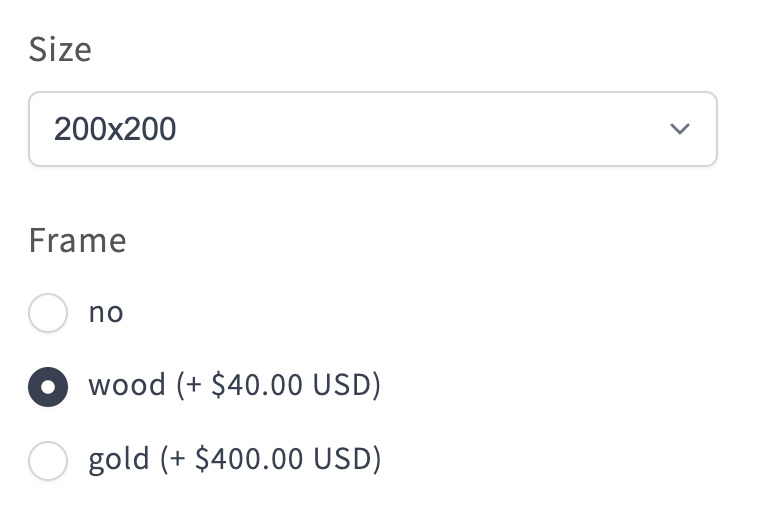#### Price based on dimension inputs with tiered pricing

This is an advanced example with tiered pricing based the provided dimension.

Create an option of type "Dimension":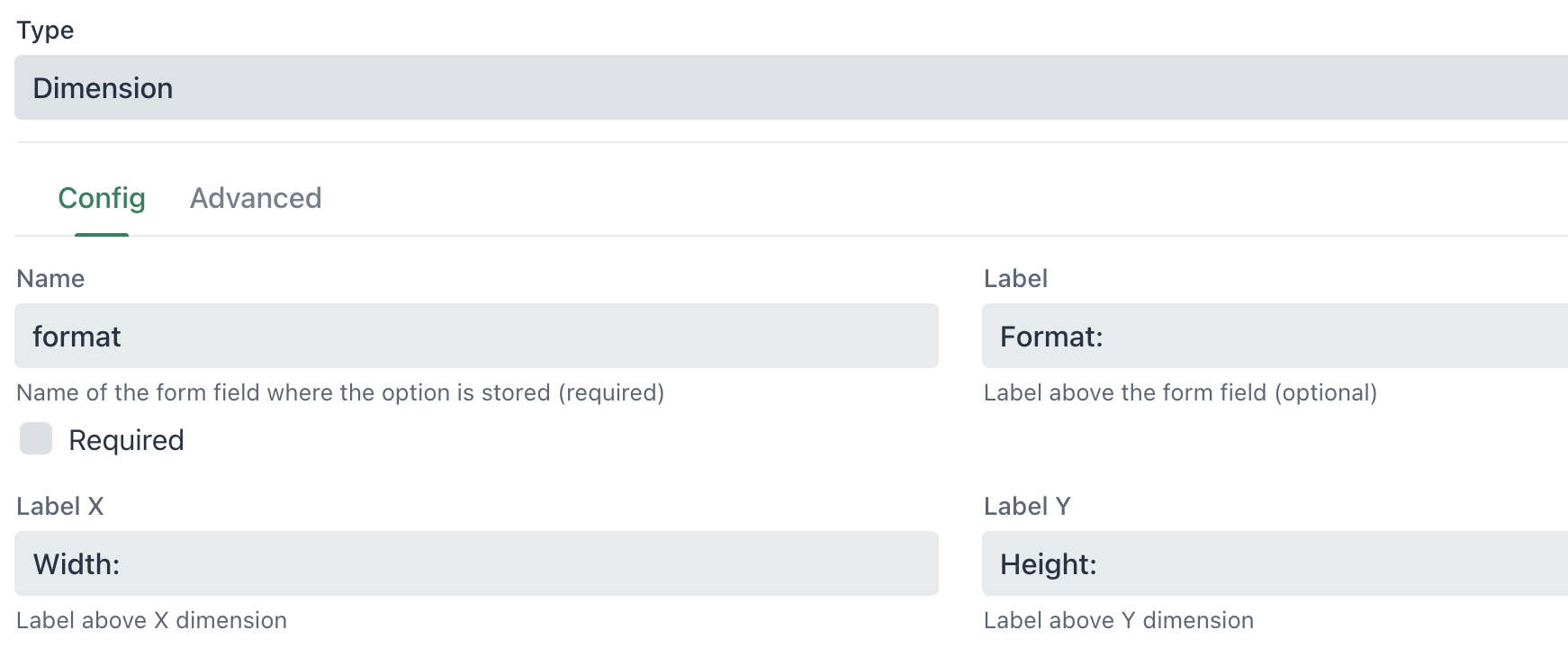In the Advanced tab of the option, it is possible to directly provide a price formula: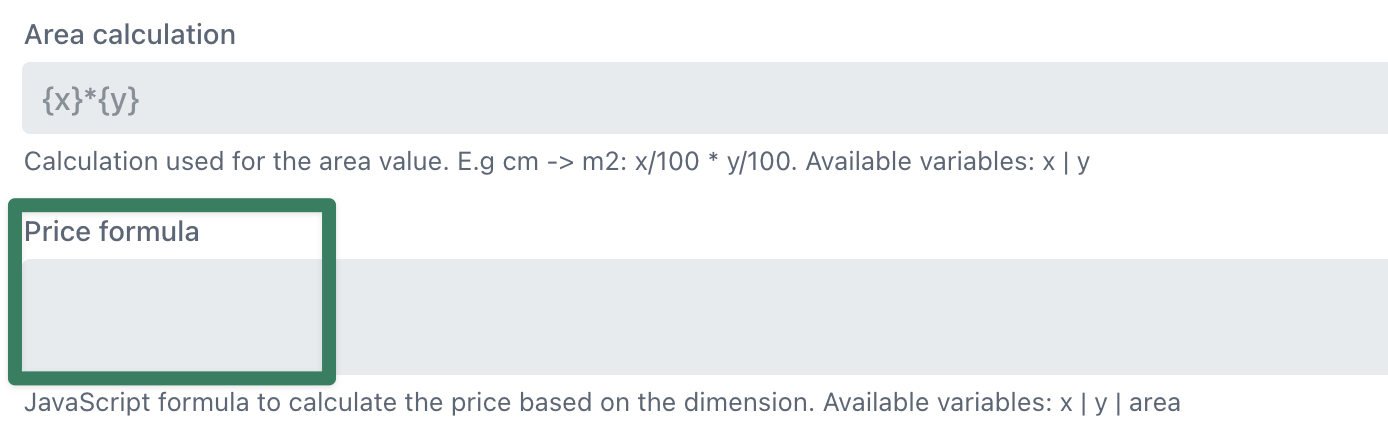The following price formula calculates a price based on the dimensions area.  The formula uses if statements to calculated tiered pricing (less per square meter the larger the area):

`// default square pricevar squarePrice = 5;console.debug('square price before', squarePrice);// larger area > lower square priceif(area > 50){  squarePrice = squarePrice * 0.5;} else if(area > 25){  squarePrice = squarePrice * 0.75;} else if(area > 10){  squarePrice = squarePrice * 0.9;}console.debug('square price after', squarePrice);// calculate pricevar priceCalc = squarePrice * area;// return the calculated pricereturn priceCalc;`

Note:  the variable area is automatically calculated by the dimension option.

##### Different materials example

The price formula can also be removed from the dimension option and instead added on another option type with more option values.

Create another option for the selection of a material: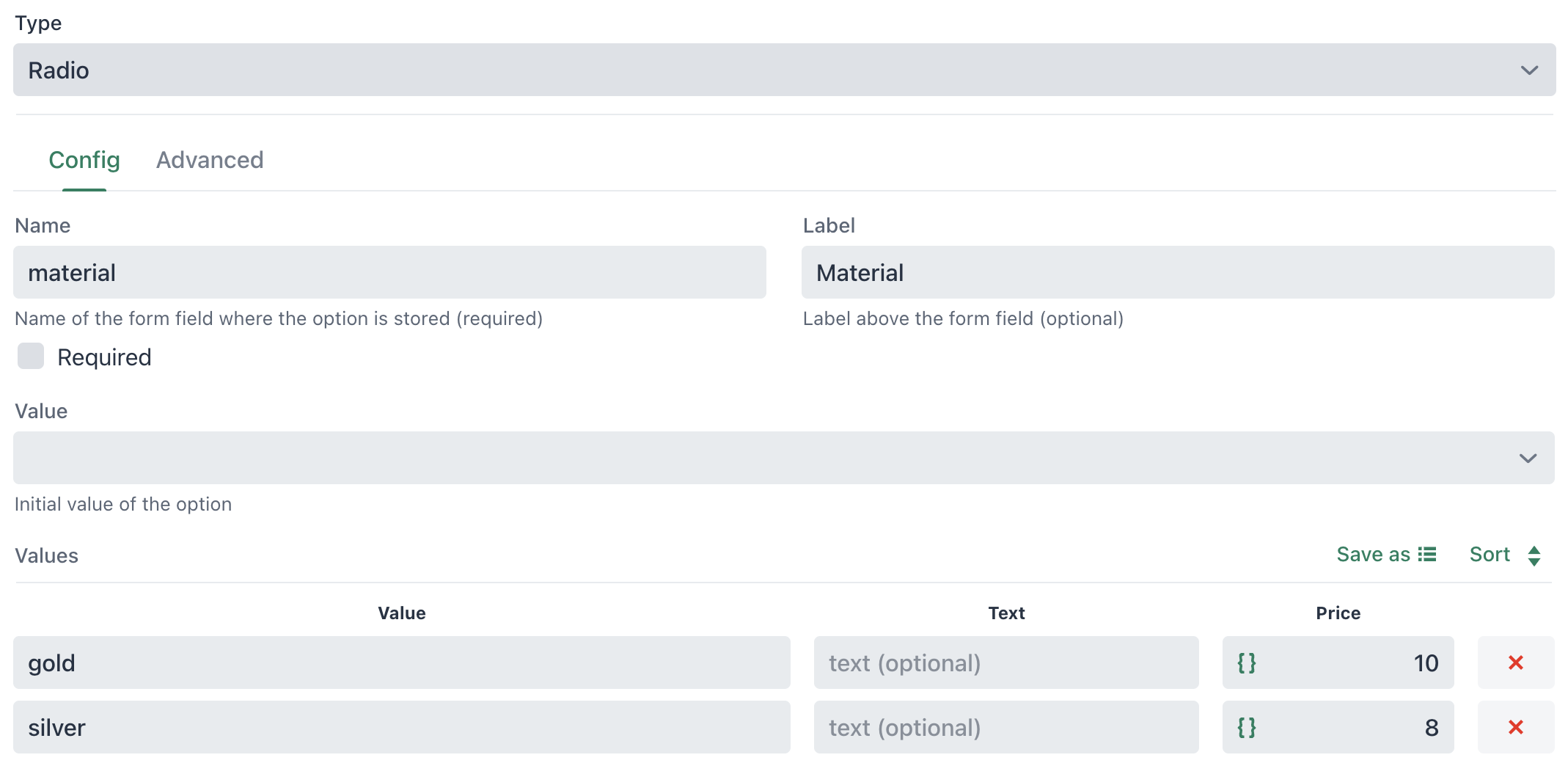`// get area from 'format' dimension option var area = options.controller('format').area ;console.debug('format area', area);// material square price (set in option.price)var squarePrice = price;console.debug('square price before', squarePrice);// larger area > lower square priceif(area > 50){  squarePrice = price * 0.5;} else if(area > 25){  squarePrice = price * 0.75;} else if(area > 10){  squarePrice = price * 0.9;}console.debug('square price after', squarePrice);// calculate pricevar priceCalc = squarePrice * area;// return the calculated pricereturn priceCalc;`

Now each option value calculates the price for the specific material and dimension: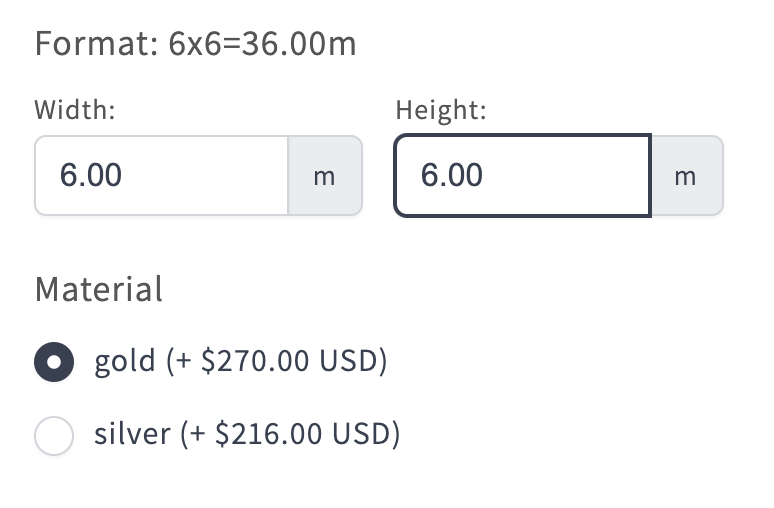#### Product variant

If you want to calculate a price based on the selected variant, you can access the current variant object using the following code:

`// get current variant object var variant = options.variant();  // get price in decimals (returned in micros)var variantPrice = variant.price / 100; // get variant title var variantTitle = variant.title;`

## Value formula

The "Value formula" option type can be used to calculate a custom option value (not the price).  This is useful if you want to have some intermediate calculation that you want to save on the order.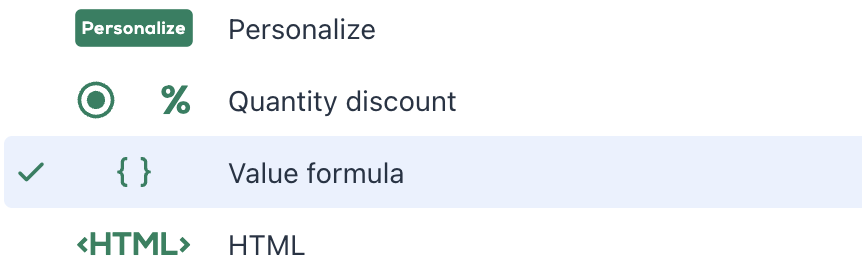#### Example

To calculate a total based on two different option inputs, you can use the following value formula:

`parseFloat(options.value('number')) + parseFloat(options.value('pages'))`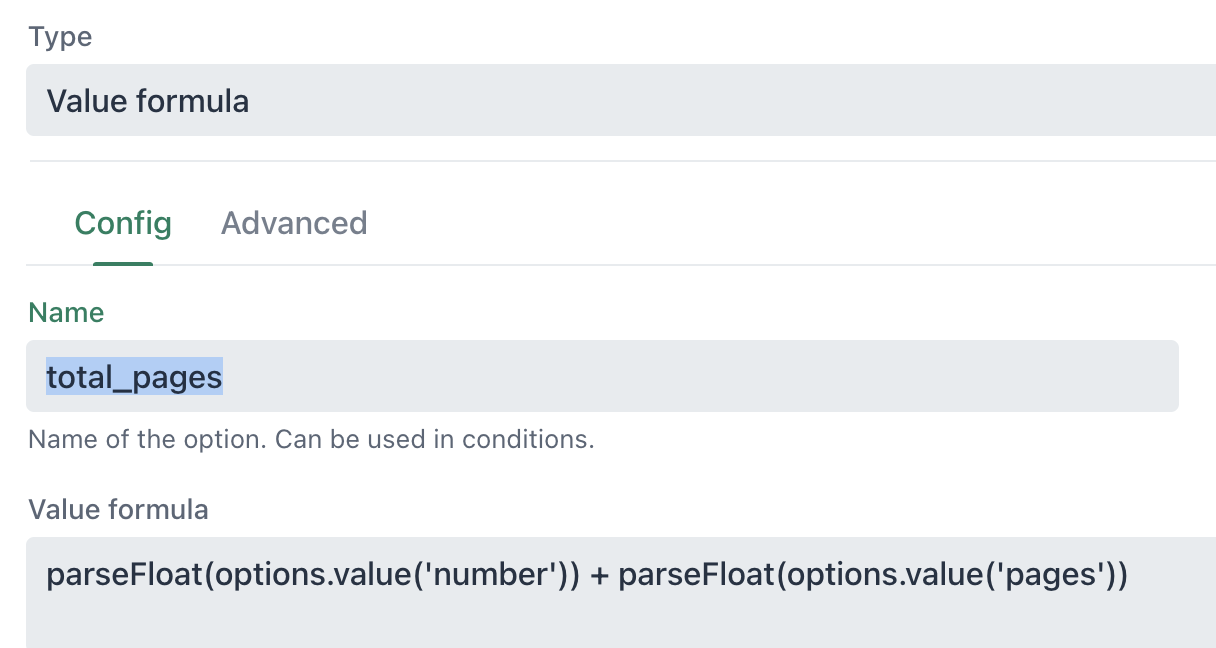Calculated values can also be used in conditions:#### Dimension X/Y conditions

If you need to add a condition on the dimension option input, you can create a value formula that just reads the x or y value of the dimension controller: (Make sure to reference the correct option name of your dimension option)

`options.controller('dimension').x`